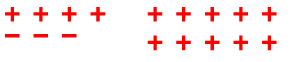### Home > CC2 > Chapter 4 > Lesson 4.1.2 > Problem4-16

4-16.

Draw a diagram that could be represented by each of the following number expressions. Then circle the terms and calculate the value of each expression. Homework Help ✎

1. $4+2(5)+(−3)$

Remember the Order of Operations when solving this kind of problem.

$4+2(5)+(−3)$
$4+2(5)+(−3)$

$4+10+(−3)$
$14+(−3)$
$11$

There are many possible diagrams for this problem.
An example is below:2. $4+2(5+(−3))$

See part (a).
Don't forget to do everything inside the parentheses first before you simplify the rest of the expression.